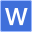﻿ 2019六年级数学寒假作业答案（11-20页）

# 2019六年级数学寒假作业答案（11-20页）

2019-2-13 17:08:00?? 无忧考网???? [ 手机版 ] [下载Word文档 ]

【导语】一声欢笑，一点轻松，多彩的寒假悄悄起程;一丝开心，一点惬意，单纯的快乐如此美丽;一分年少，一点激昂，青春的生活从不迷茫。无忧考网准备了以下内容，供大家参考。6)(1)2+2=2×2或0+0=0×0

(2)①4/15，4/15②9/70，9/70

③25/66，25/66④36/91，36/91

(3)4，7，3/7，3/7，

2/3×2/5=2/3-2/5(此小题答案多种)

7)(1)21÷7/17=51(枚)

(2)8÷(1-3/4)=8×4=32(千克)

(3)750÷(1-7/32)=750÷32/25=960(米)

(4)96-45=51(页)

明明：96×3/4-15=72-15=72-15=57(页)≠51(页)

聪聪：96×1/2+3=48+3=51(页)=51(页)

答：聪聪说得对.

提高篇：

(1)原式=2010/2011×(2011+1)=2010+2010/2011=2010又2010/2011(对不起，打出的分数在博文中显现不出来，只能这样打了)

(2)原式=63/2×2/3+124/3×3/4+205/4×4/5=21+31+41=93

(3)原式=3/2×4/3×5/4×……×100/99×101/100=101/2(2)(42-24)÷1/4=18×4=72

(3)18÷3/5-18=30-18=12(人)

(4)(2/5÷4)×(2/5÷4)=1/10×1/10=1/100(平方米)

8)(1)5.4÷(1+8)=5.4÷9=0.6(千克)

0.6×8=4.8(千克)

(2)360×(1-1/3)=240(只)5+3=8(份)

黑兔：240×5/8=150(只)灰兔：240×3/8=90(只)白兔：360×1/3=120(只)

(3)9，36，12

提高篇

1)(1)7：9(2)2：3：5(3)3：4：10

2)梨的质量是橘子的2/5，说明梨和橘子质量的比是2：5

则梨、橘子和苹果质量的比是2：5：6，即三种水果共分成2+5+6=13(份)梨占2/13，所以梨有260×2/13=40(千克)(5)3：4

(6)1/51/4

(7)7/6

4)80+60+100=240(千瓦时)

小明家：120×80/240=40(元)小红家：120×60/240=30(元)

小华家：120×100/240=50(元)

5)X=5/2X=1/18

6)答：这个比与教科书中的比不同.体育比赛中只是记载得分情况.

7)(1)1+2+3=6(份)

低年级：360×1/6=60(本)

中年级：360×2/6=120(本)

高年级：360×3/6=180(本)

(2)2+3=5(份)3/5×2/5=6/25(千克)

(3)①0.5×1000=500(千克)

②1000+1=1001药液：160.16×1/1001=0.16(千克)

(4)5+3=8(份)白昼：24×5/8=15(小时)24×3/8=9(小时)

(5)350×(1-1/7)=350×6/7=300(千米/时)

(6)解：设这条水渠全长X千米。

X-1/6×X-3/5×X=7/8

7/30X=7/8

7/30X÷7/30=7/8÷7/30

X=15/4

答：这条水渠全长15/4千米。

提高篇

1)第二天看了全书的8/15×3/4=2/5

两天共看了全书的8/15+2/5=14/15

答：小华没有看完这本书，因为小华两天共看了全书的14/15，小于1。

2)第二次用去整桶油的(1-1/5)×1/2=4/5×1/2=2/5

解：设这桶汽油原有X千克。

1/5X+2/5X=24

3/5X=24

3/5X÷3/5=24÷3/5

X=40

答：这桶汽油原有40千克。